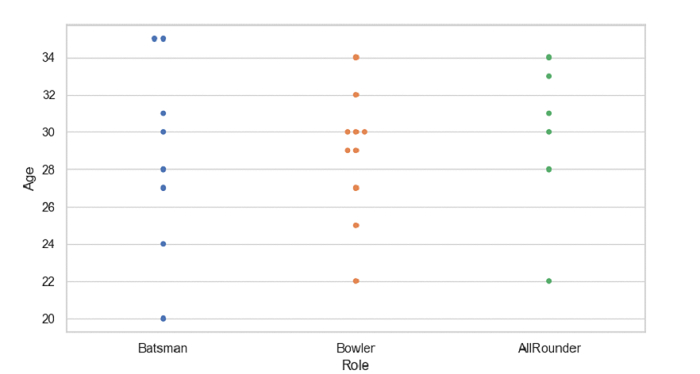# Python Pandas - Group the swarms by a categorical variable with Seaborn

Swarm Plot in Seaborn is used to draw a categorical scatterplot with non-overlapping points. The seaborn.swarmplot() is used for this. Group the swarms by a categorical variable by simply setting it as one of the x and y coordinates.

Let’s say the following is our dataset in the form of a CSV file − Cricketers2.csv

At first, import the required libraries −

import seaborn as sb
import pandas as pd
import matplotlib.pyplot as plt

Load data from a CSV file into a Pandas DataFrame −

dataFrame = pd.read_csv("C:\Users\amit_\Desktop\Cricketers2.csv")


Group the swarms by a categorical variable −

sb.swarmplot(x = dataFrame["Role"], y = dataFrame["Age"])

## Example

Following is the code −

import seaborn as sb
import pandas as pd
import matplotlib.pyplot as plt

# Load data from a CSV file into a Pandas DataFrame

# set the theme
sb.set_theme(style="whitegrid")

# group the swarms by a categorical variable
sb.swarmplot(x = dataFrame["Role"], y = dataFrame["Age"])

# display
plt.show()

## Output

This will produce the following output −Updated on: 04-Oct-2021

70 Views